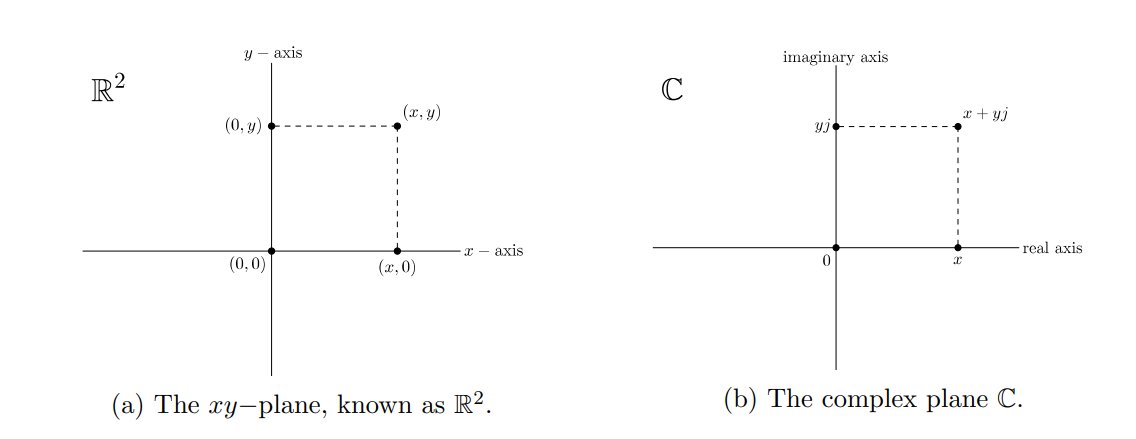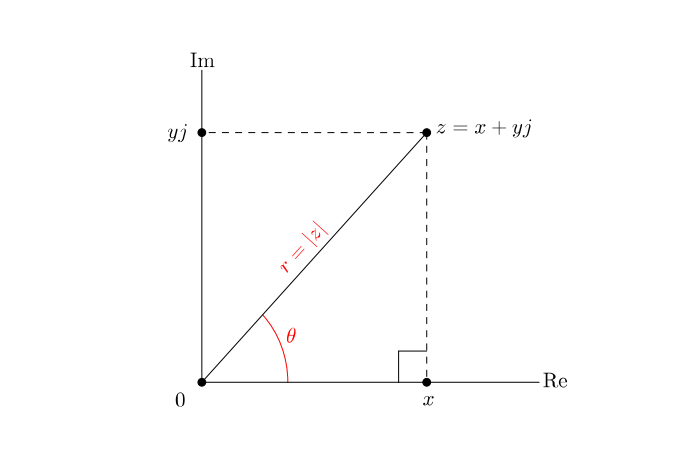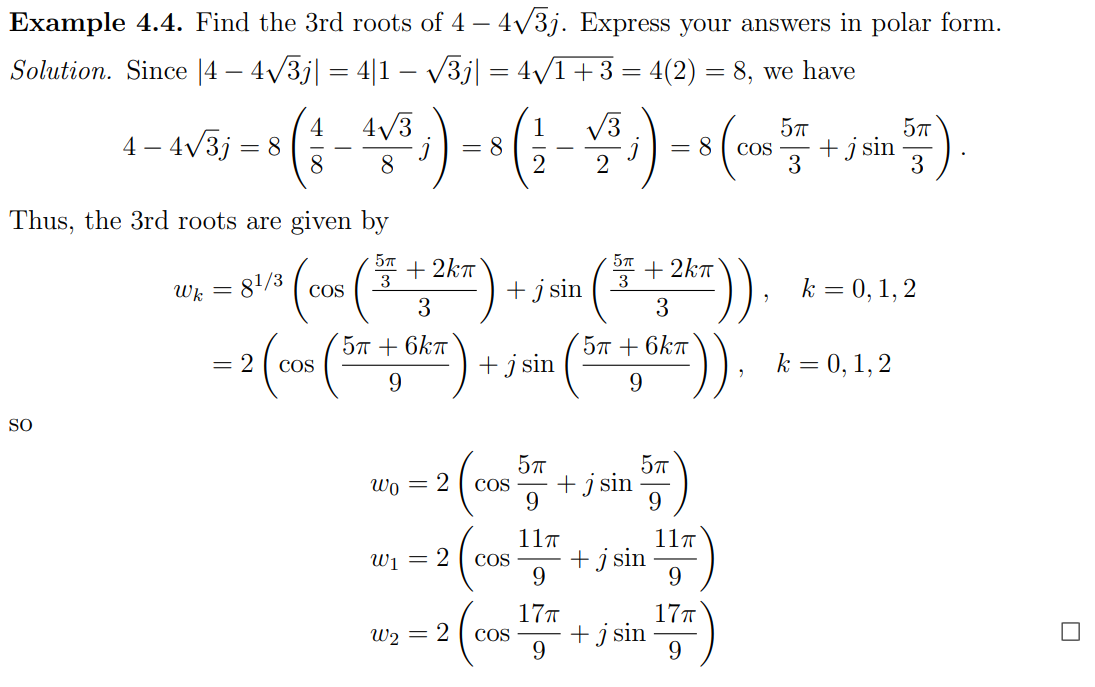# Complex Number §

Three ways to represent a complex number.

### Standard Form §

Definition: A complex number in standard form is an expression of the form where and satisfies . The set of all complex numbers is denoted by

title:
Note that I learned to use $j$ rather than $i$ since  that letter is used in the modelling of electric networks.
$i$ is NOT equal to $\sqrt{-1}$. If it were, then
$$-1 = i^2 = i \cdot i = \sqrt{-1} \cdot\sqrt{-1} = \sqrt{-1 \cdot -1 } = \sqrt{1} = 1$$

Let with .

### Inverse of Complex Number §

For , , exists if and only if . Besides, when it exists,

### Conjugate §

The complex conjugate of with is

Properties of Conjugates. Let with where . Then

### Modulus §

The modulus of with is the nonnegative real number

The modulus is basically the absolute value if we are dealing with real numbers, and this is actually where the modulus comes from. 

Properties of Modulus. Let . Then

### Geometry of Graphically §### Polar Form §

There is another way we can represent imaginary numbers, which will be super useful for complex multiplication and division.

We invent this plot, where the x-axis are the Real numbers and y-axis are the imaginary numbers.We see from the above image that

The polar form of a complex number is given by where and is an argument of .

title: Polar Form: Use $z=r\angle\theta$
When I first learned polar form in [[Linear Algebra|MATH115]], we always wrote it as $z = r(\cos\theta+j\sin\theta)$, but this is super long. Instead, just use
$$z = r\angle\theta$$
which provides the same amount of information in a very concise way.
###### Exercise: Write in polar form. §

Since we have and , we know that Answer:

### Polar Multiplication and Division §

Multiplication Division

The MATH115 notation…

### Powers of Complex Numbers §

de Moivre’s Theorem. If , then for any .

### Complex nth Roots §

Let be nonzero, and let n be a positive integer. Then the n distinct nth roots of z are given by for### Complex Exponential Form §

Exponential form is really cool, because we can actually start relating exponentials with sins and angles!

Derived from Euler Formula, the complex exponential form of is given by

### Complex Polynomials §

Theorem 5.2. Let be a real polynomial. If is a root of , then so too is .